Contents

2.2. Generalization

Generalization is one of the central problems of supercompilation. It breaks down naturally in two parts: (1) a decision to generalize the current configuration C with some of its predecessors C' trying to reduce C to it; we need a `whistle' to warn us that if we do not try reduction, we may end up with infinite driving; and (2) the generalization proper, i.e., defining a configuration Cgen such that both C and C' are its subsets. A good generalization algorithm must find a balance between two extreme cases: a too willing generalization, which makes the resulting program interpretive and leaves it unoptimized; and a generalization postponed for too long so that the supercompilation process never ends.

In  I defined an algorithm of whistling for lazy supercompilation of nested function calls, and proved its termination. This algorithm was implemented in SCP-2, and it workes very well, but only within its domain of usefulness.

It works with stacks representing nested function calls, and refers to an unspecified algorithm for generalization of flat configurations, i.e., ones without nested function calls.

In SCP-2 and SCP-3 we used several empirically found algorithms of generalizing flat expressions, but no termination theorems were proven.

Sørensen and Glück,  used the Higman-Kruskal theorem about homeomorphic embedding (HK for short) to define a whistle for supercompilation which is of a proven termination. The data domain in  is the set of functional terms with fixed arity of each functional symbol. This domain is sufficient for representation of lists, but not Refal expressions. Refal allows concatenation of terms, which can be seen as the use of a functional symbol of arbitrary arity (a varyadic symbol). Fortunately, the Higman-Kruskal theorem allows varyadic symbols. When I learned this, I defined a whistle for supercompilation in Refal along the lines of .

I will briefly outline the concept of embedding following the work by Dershowitz .

Let a finite set F of functional symbols be given. Consider the set T(F) of all functional termswith functional symbolsF. Some symbols may be of arity n= 0; they are constants, and we shall write them without parentheses: f for f( ). Some of the symbols may be varyadic: different n in different calls.

Definition. The homeomorphic embedding relation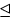on a set T(F) of terms is defined recursively as follows:if either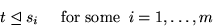or

f=g   and   tj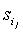for all     j = 1,2,...,n.

where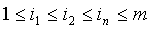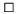Note that in the second rule f and g must be identical, but their calls may have different number of terms:; some of the terms in f may be ignored.

Theorem. (Higman,Kruskal) If F is a finite set of function symbols, then any infinite sequenceof terms in the set T(F) of terms over F contains two terms tj and tk, where j<k, such that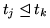To use HK, we map the set of all Refal terms TR onto the domain of functional terms T(F) over some set F of functional symbols.

Definition. The set F is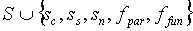, where S is the set of Refal symbols except numbers; it is finite because only those non-numerical symbols that enter the program can appear in computation. Symbols from S, as well as the two special symbols se and ss, are of arity 0, while the other two symbols are varyadic. The mapping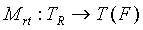is recursively defined by Table 1, where s stands for any non-numerical symbol, n is any number, t is a term, and e is an expression.Table 1: The mapping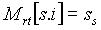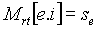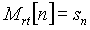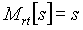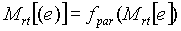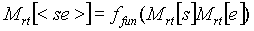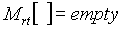In Refal the most general data structure is that of expressions, not terms. But it is easy to reduce a relation on expressions to a relation on terms: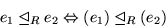We define the homeomorphic embedding relationon Refal terms as the mapping of the relation: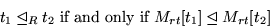Rewriting the definition ofin terms of Refal, we have:

Definition. The homeomorphic embedding relationon the set TR holds if either term is embedded in term:(1)

whereand ei for i=1,2 are some expressions; or expression is embedded in expression: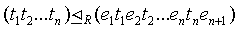(2)

wherefor i = 1,...,n, and any of the expressions ei may be empty.This definition translates into the algorithm in Refal given in Table 2.

 Table 2: The Refal program for the embedding relation*   results   in   T   if   t1t2, * and in F otherwise. Emb  {eX  =  >  } * Decide if the second case must be considered Dec  {  eX  T  =   T ; eX  F  =  F ; eX  2  =   } Embk  { sN  s1  s1   =  T ; sN  t1    s2  =  F ; sN  ()   (e1)  =  T ; 1  t1  ()   =  2 ; 2  t1  ()   =  F ; 3  t1  (t2   e3) , < Emb  t1  t2> : {   T  =  T ; F   =    } ; 2  ( t1 e2) (t.1s   e.2s) ,  : { T  =  ; F   =    } ; }

This whistle was not yet tested in the computer, but I believe it will work well.

I discovered, with some surprise, that the embedding relation on Refal expresions leads to a whistle different from that derived from the embedding relation on Lisp's lists, even though these two kinds of symbolic objects may look identical. I cannot go into detail here (see  for that). Just an example and a few words.

Consider this relation: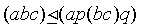If it is understood as a relation between Refal expressions, it does not hold. But if we see it as a relation between lists, it does hold. Moreover, the algorithm for Refal expressions above is linear with the size of expressions, while the corresponding recursive algorithm for lists is exponential (it can be converted, though, into a quadratic iterative algorithm). I must also notice that we can have the Refal whistle working with lists by exploiting a one-to-one mapping between these two domains.

A few words about generalization proper. With a given whistle algorithm, various algorithms of generalization proper may be used. For our whistle algorithm all variables of a given type (s or e) are the same, while for generalization proper this is not so, of course. Still the embedding relation which caused the whistle may serve as a starting point for generalization. In particular, if the relationin the case expression embedded in expression happens, for some i, to be an equality, we can leave it as a common part in generalization. For example, the embedding:

a  b cp  a  b  c  q  r

gen[a b c, p a b c q r] = x1 a b c x2

where x1 and x2 are some e-variables. This method, though, works only for Refal expressions, not for lists. With lists as the basic data structures, generalization can preserve only that common substructure which is on the left, but not on the right side. Even though a list,  such as (a b c), looks like a string, it is, in fact, a binary tree which in the Refal representation is

(a(b(c nil)))

We can generalize it with a list which extends it on the right side, without losing the common part:

gen[ (a(b(c nil))), (a(b(c(p nil)))) = (a(b(c x1)))

but if the extension is on the left, the most specific generalization is a free variable. The common part is lost:

gen[ (a(b(c nil))), (p(a(b(c nil)))) ] = x1

Unfortunately, when a program works by iterations (as opposed to programs where data is passed from the result of one function to the argument of another) it is exactly on the left side that the lists are growing. Because of this, a supercompiler working with lists may not perform partial evaluation in cases where a Refal supercompiler easily does it.

Contents  Next